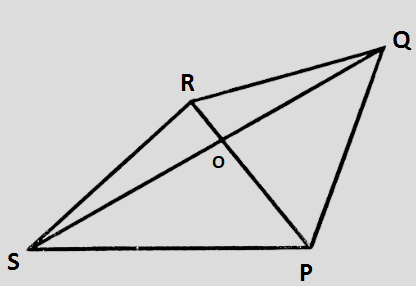# Draw a rough sketch of a quadrilateral PQRS. Draw its diagonals. Name them.Is the meeting point of the diagonals in the interior or exterior of the quadrilateral?

To do:

We have to draw a rough sketch of a quadrilateral PQRS and name its diagonals.

Solution:

Given below is a quadrilateral PQRS.

$RP$ and $QS$ are its diagonals. The diagonals $RP$ and $QS$ intersect at a point that lies in the interior of the quadrilateral $PQRS$.